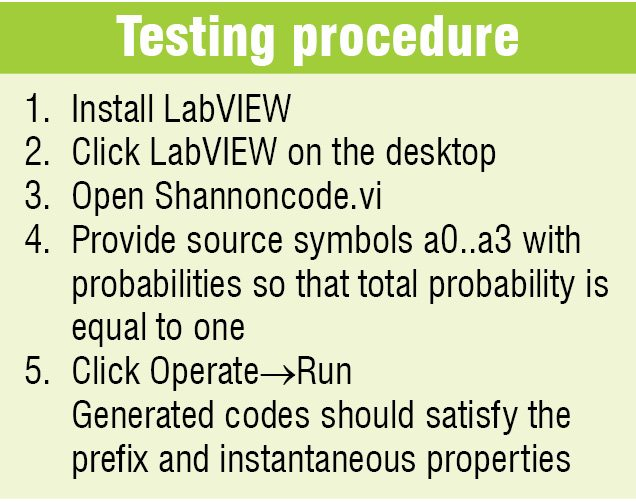# Implementation Of Shannon Encoding Algorithm Using LabVIEW (Part 2)

6628

Unbundle-by-Name function. It returns the cluster elements whose names you specify as shown in Fig. 6. Input cluster is the cluster whose elements you want to access and element 0..m-1 is the element of input cluster called 0..m-1.

We do not have to keep track of the order of the elements within the cluster. This function does not require the number of elements to match the number in the cluster. After you wire a cluster to this function, you can select individual elements from the function.

Logarithm Base 2 function. It computes base 2 logarithm of x. If x is 0, log2(x) is negative infinity as shown in Fig. 7. Here, x can be a scalar number, array or cluster of numbers, array of clusters of numbers and so on. log2(x) is of the same numeric representation as x.

Bundle function. If x is not complex and is less than 0, log2(x) is NaN (not a number).

It assembles a cluster from individual elements as shown in Fig. 8. The cluster is one whose value you want to change and element 0..n-1 accepts any data type, and output cluster is the resulting cluster.

You can also use this function to change the values of individual elements in an existing cluster without having to specify new values for all elements. To do so, wire the cluster you want to change to the middle cluster terminal of this function. When you wire a cluster to this function, function resizes automatically to display inputs for each element in the cluster.

Quotient and Remainder function. Computes integer quotient and remainder of the two inputs as shown in Fig. 9. x and y can be scalar numbers; x-y*floor(x/y) is the remainder and floor(x/y) is the integer quotient. This function rounds floor(x/y) to the nearest integer towards infinity.

Clusters. Clusters group data elements of mixed types as shown in Fig. 10. A cluster is similar to a record or struct in text based programming languages. An example of a cluster is LabVIEW error cluster, which combines a Boolean value, a numeric value and a string.